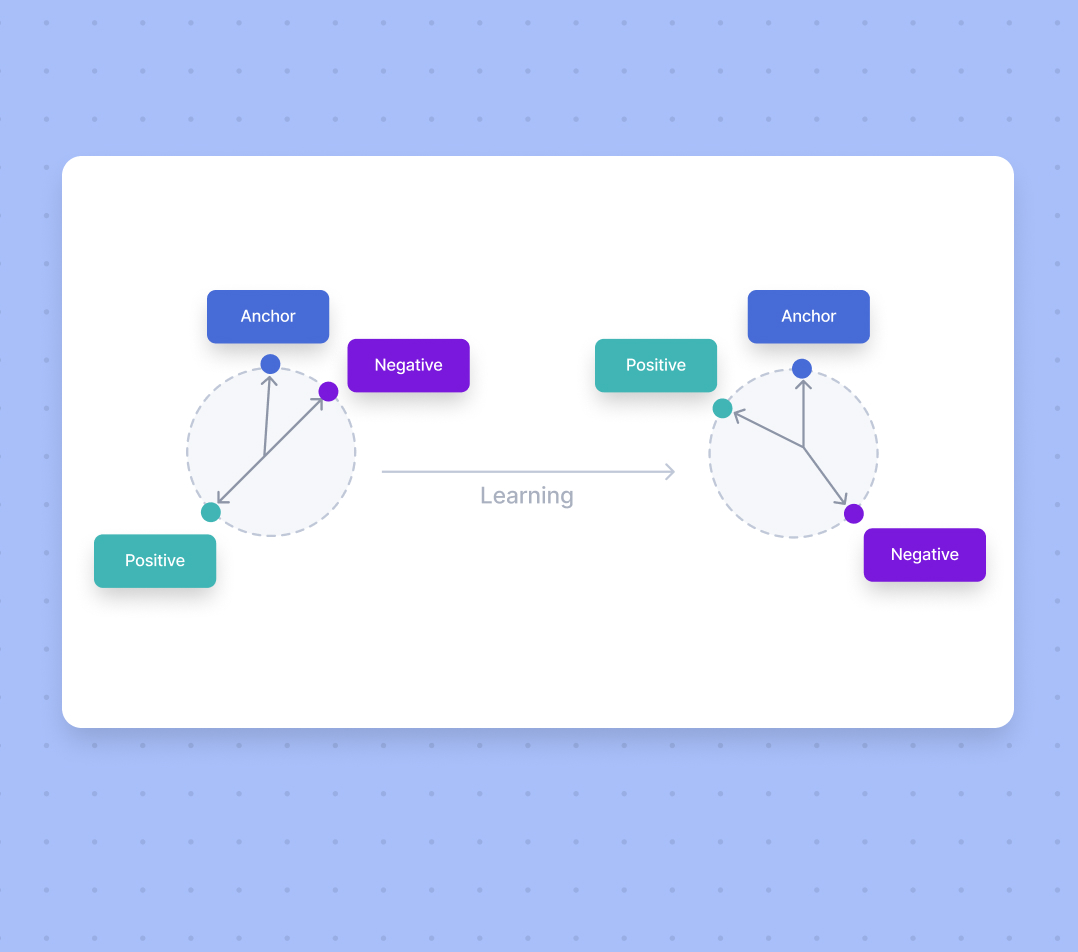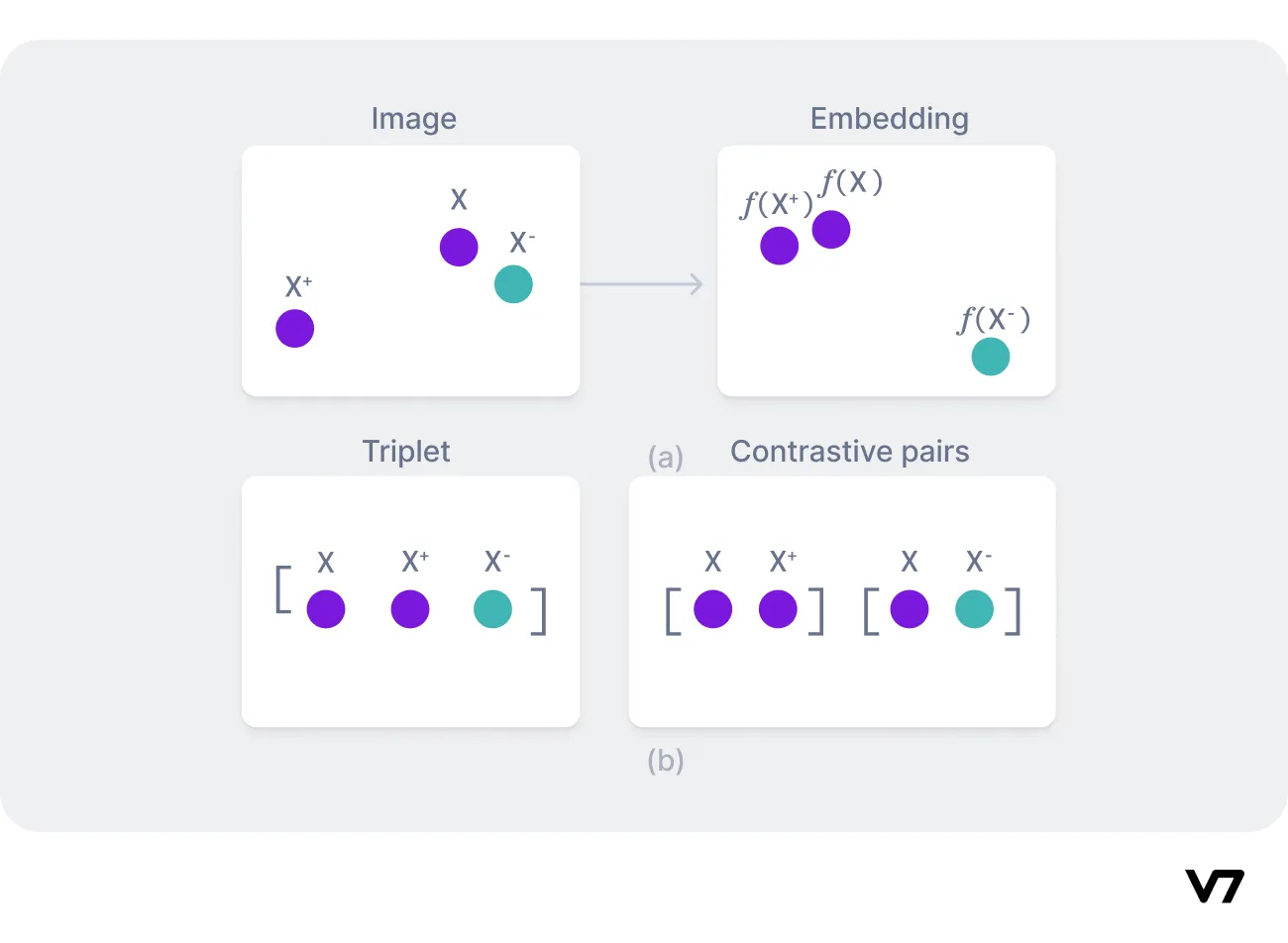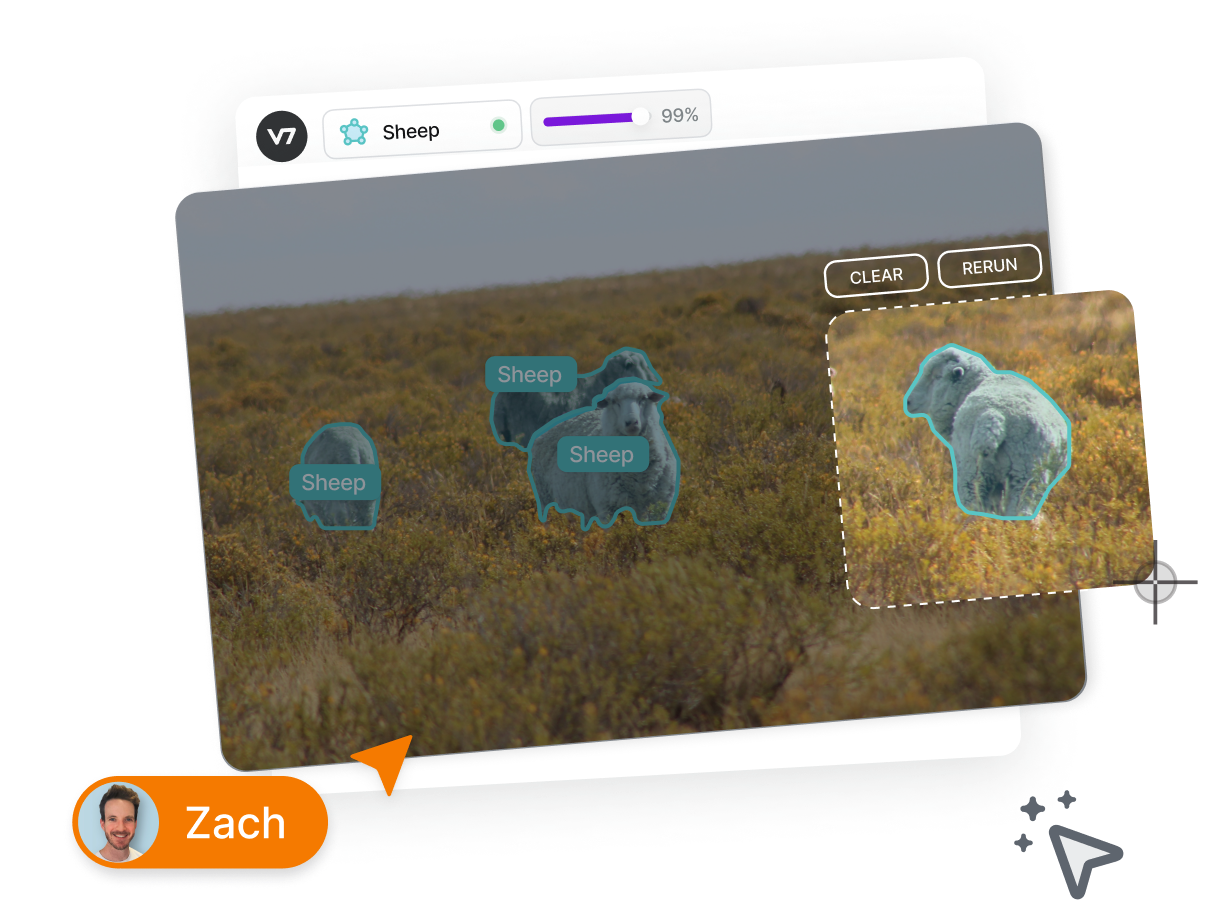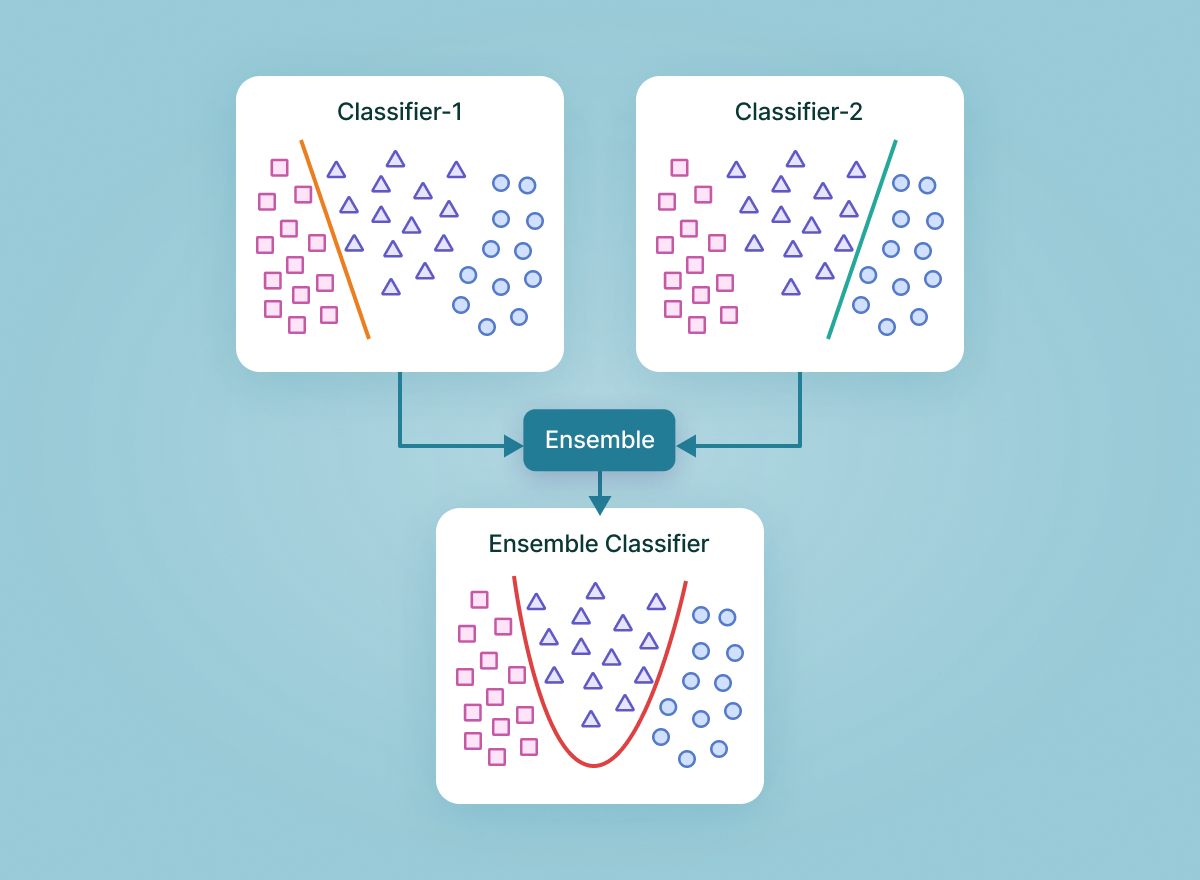# Triplet Loss: Intro, Implementation, Use Cases

What is triplet loss, how to implement it in your projects, and what are its most prominent real-world applications? Let's find out.15
April 14, 2023Real-world industry applications of machine learning, such as facial recognition software, object recognition, POS tagger, or report ranking in NLP,  still pose many multiclassification challenges.

Often, they require a solution in which the model can work for more than a million classes, and easily recognize and evaluate the similarity and dissimilarities.

A paper called FaceNet: A Unified Embedding for Face Detection and Clustering introduced triplet loss in 2015 with the goal of tackling this issue. It has since evolved into one of the most prominent loss functions for supervised similarity and metric learning.

Triplet loss forces the separation between different pairings by a specified margin value, where related data points are projected near each other. In contrast, disparate data points are projected far away.

Here’s what we’ll cover:

Train ML models and solve any computer vision task faster with V7.

Don't start empty-handed. Explore our repository of 500+ open datasets and test-drive V7's tools.

Or, are you ready to jump straight into building your models? Check out:

## What is triplet loss?

Triplet loss is a way to teach a machine-learning model how to recognize the similarity or differences between items. It uses groups of three items, called triplets, which consist of an anchor item, a similar item (positive), and a dissimilar item (negative).

The goal is to make the model understand that the anchor is closer to the positive than the negative item. This helps the model distinguish between similar and dissimilar items more effectively.

In face recognition, for example, the model compares two unfamiliar faces and determines if they belong to the same person.

This scenario uses triplet loss to learn embeddings for every face. Faces from the same individual should be close together again and form well-separated clusters in the embedding space.

The objective of triplet loss is to build a representation space where the gap between similar samples is smaller than between different examples. By enforcing the order of distances, triplet loss models are embedded so that samples with identical labels appear nearer than those with other labels.

Hence, the triplet loss architecture helps us learn distributed embedding through the concept of similarity and dissimilarity. The mathematical depiction is shown below:

$∑iN||f(xia)-f(xip)||22-||f(xia)-f(xin)||22+∝$

Where

• f(x) accepts an input of x and generates a 128-dimensional vector w
• I represents the i'th input
• The subscript a denotes an anchor image, p is a positive image, and n is a negative image
• $\propto$ refers to the bias

The goal is to minimize the above equation by minimizing the first term and maximizing the second term, and bias acts as a threshold.

An anchor (with fixed identity) negative is an image that doesn’t share the class with the anchor—so, with a greater distance. In contrast, a positive is a point closer to the anchor, displaying a similar image. The model attempts to diminish the difference between similar classes while increasing the difference between different classes.

### Triplet loss vs. contrastive loss(a) Distance metric learning (b) Triplet loss and contrastive loss architecture

Although both triplet loss and contrastive loss are loss functions used in siamese networks—deep learning models for measuring the similarity of two inputs—they have particular distinctions.

The critical distinction between triplet and contrastive loss is how similarity is defined and the number of samples used to compute the loss. The following pointers indicate the key differences.

Input: The number of inputs used to compute the loss differs. Triplet loss requires three inputs (anchor, positive, and negative), whereas contrastive loss requires only two (positive and negative) inputs.

Distance: The goal of triplet loss is to minimize the distance between the anchor and the positive example while raising the gap between the anchor and the negative example. The purpose of contrastive loss is to minimize the distance between the positive (similar) examples while increasing the distance between the negative (dissimilar) examples

Use cases: Triplet loss is used in problems that aim to acquire a representation space where similar cases are close together, and different examples are far apart—such as facial recognition. Contrastive loss is commonly employed in applications such as picture categorization.

Sensitivity: The margin parameter specifies the minimum distance that has to be kept between the anchor and the positive example and the maximum distance that has to be retained between both the anchor and the negative example, which is more dependent upon the selection of triplet loss. The margin parameter has less of an effect on contrast loss.

##### 💡 Read more: The Beginner’s Guide to Contrastive Learning
Label 10x faster with auto-annotate

Pixel-perfect annotation quality, increased efficiency, and lowered overheads.## What is triplet mining?

With the triplet loss objective of analyzing the distance via a margin parameter, sometimes all triplets may be less relevant for training the model.

If the algorithm is trained with excessive "easy" triplets, the model may gravitate to a suboptimal output that does not generalize well.

In contrast, the training process could be weak and inefficient if the model has been trained on excessive "hard" triplets, in which the anchor and positive instances are already close.

### Triplet categories

Triplets can be classified into three categories based on the distance between the anchor, positive, and negative samples.

#### Hard negatives

Hard negatives are the negative samples closest to the anchor. These samples are challenging for the model to distinguish and are the most informative for training. They require the model to learn more complex and discriminative features to differentiate between the anchor and the negative samples.

#### Semi-hard negatives

Semi-hard negatives are negative samples farther from the anchor than the positive sample but still have a positive loss. These samples are easier for the model to distinguish than hard negatives but are still useful for training.

#### Easy negatives

Easy negatives are negative samples the furthest from the anchor. These samples are too easy for the model to distinguish and do not provide useful training information.

Triplet mining aims to pick informative triplets that contribute to successful learning by selecting "hard" triplets that are difficult for the model to correctly categorize and avoiding "easy" triplets that the model can accurately classify. Informative triplets here consist of training examples chosen to improve the model's performance in triplet loss training.

Triplet mining can be done in numerous ways, notably:

• batch hard triplet mining— involves computing the triplet loss only for the hardest negative sample for each anchor-positive pair in a batch.
• batch all triplet mining—involves computing the triplet loss for all possible combinations of anchor, positive, and negative samples in a batch.
• semi-hard triplet mining—involves selecting triplets where the negative sample is closer to the anchor than the positive sample but still within the margin. The margin is a predefined constant representing the minimum acceptable distance between the anchor-positive and the anchor-negative pair. This allows the model to focus on learning from challenging but not too difficult examples.
• distance-weighted triplet mining—the main idea is to select triplets by weighting the probability of choosing a particular triplet based on the distances between the anchor, positive, and negative samples. This approach encourages the model to focus on a broader range of examples during training, including easy, semi-hard, and hard triplets, rather than just semi-hard ones.

The online and offline strategies are two approaches for selecting triplets for training.

### Online triplet mining

Online triplet mining is a deep learning technique that dynamically generates triplets of data points (anchor, positive, and negative) during training. It selects the hard triplets from similar anchor and positive samples. This method may enhance the training phase by lowering the amount of non-learning triplets and picking the most difficult samples to optimize the model.

Online triplet mining is important in training siamese networks using triplet loss. It ensures the model has been trained on informative triplets, contributing to good learning and generalization. The model learns to differentiate between similar and dissimilar examples and generalize to new, unknown data by picking informative triplets during training.

The online triplet model has several advantages, including enhanced model performance, reduced training time for selecting the hardest triplets, and adaptability because they are dynamically picked based on the model's current state. However, it’s computationally expensive, sensitive to batch size and margin hyperparameters, and prone to overfitting.

### Offline triplet mining

Offline triplet mining is a deep learning method that produces triplets (anchor, positive, and negative) of data points before training. It involves the selection of all possible triplets from a dataset and eliminating those that are either overly simple or too tough for the model to learn. The remaining triplets are then used to train the model

As the triplets are chosen only once and subsequently reused throughout the training process, offline triplet mining can be more computationally effective. It also offers greater stability than online mining because the triplets are computed and fixed before training, making it less likely to overfit or underfit the training data. It’s also easier to implement, therefore, more accessible to researchers and practitioners without large-scale computational resources.

The disadvantages of offline triplet mining include a higher memory footprint—all possible triplets are loaded in memory, making it unfit for larger datasets. Additionally, the model cannot adjust to changes in data distribution and may occasionally fail to identify informative triplets, resulting in poor results due to precomputation.

The trade-offs between these advantages and disadvantages should be carefully considered when determining the triplet mining technique.

## How to implement triplet loss?

Let’s learn how to implement triplet loss step-by-step using PyTorch.

### Compute the distance matrix

The first step in implementing triplet loss is to compute the distance matrix between the anchor samples, positive samples, and negative samples.

We can use the Euclidean distance as the distance metric. Here is some sample code to compute the distance matrix:

import torch

def euclidean_distance(x, y):
"""
Compute Euclidean distance between two tensors.
"""

def compute_distance_matrix(anchor, positive, negative):
"""
Compute distance matrix between anchor, positive, and negative samples.
"""
distance_matrix = torch.zeros(anchor.size(0), 3)
distance_matrix[:, 0] = euclidean_distance(anchor, anchor)
distance_matrix[:, 1] = euclidean_distance(anchor, positive)
distance_matrix[:, 2] = euclidean_distance(anchor, negative)
return distance_matrix


In this code snippet, we define a function euclidean_distance to compute the Euclidean distance between two tensors.

We then define a function compute_distance_matrix that takes in anchor, positive, and negative samples and computes the distance matrix between them.

The distance matrix is a tensor of size (batch_size, 3). The first column contains the distances between anchor samples, the second column contains the distances between the anchor and positive samples, and the third column contains the distances between anchor and negative samples.

### Batch all strategy

Here is the sample code to implement the batch all strategy:

import torch.nn.functional as F

def batch_all_triplet_loss(anchor, positive, negative, margin=0.2):
"""
Compute triplet loss using the batch all strategy.
"""
distance_matrix = compute_distance_matrix(anchor, positive, negative)
loss = torch.max(torch.tensor(0.0), distance_matrix[:, 0] - distance_matrix[:, 1] + margin)
loss += torch.max(torch.tensor(0.0), distance_matrix[:, 0] - distance_matrix[:, 2] + margin)


In this code snippet, we define a function batch_all_triplet_loss that takes in anchor, positive, and negative samples and computes the triplet loss using the batch all strategy. The margin parameter controls the minimum distance between the anchor and negative samples.

### Batch hard strategy

Here is the sample code to implement the batch-hard strategy:

import torch

def batch_hard_triplet_loss(anchor, positive, negative, margin=0.2):
"""
Compute triplet loss using the batch hard strategy.
"""
distance_matrix = compute_distance_matrix(anchor, positive, negative)
hard_negative = torch.argmax(distance_matrix[:, 2])
loss = torch.max(torch.tensor(0.0), distance_matrix[:, 0] - distance_matrix[:, 1] + margin)
loss += torch.max(torch.tensor(0.0), distance_matrix[:, 0][hard_negative] - distance_matrix[:, 2] + margin)


This code snippet implements the batch-hard strategy for computing the triplet loss. The function batch_hard_triplet_loss takes in anchor, positive, and negative samples and the margin parameter that controls the minimum distance between the anchor and negative samples.

First, the function computes the distance matrix between the anchor, positive, and negative samples using the compute_distance_matrix function. Then, it finds the index of the hardest negative sample by selecting the index of the negative sample with the highest distance from the anchor. This is done using the torch.argmax function on the third column of the distance matrix.

Then, the function computes the triplet loss using the formula:

max(d(a,p) - d(a,n) + margin, 0) + max(d(a, n_hard) - d(a,p) + margin, 0)

where d(a, b) represents the Euclidean distance between samples a and b.

The first term in the loss is the same as in the batch all strategy, which aims to minimize the distance between the anchor and positive samples and maximize the distance between the anchor and negative samples.

The second term focuses only on the hardest negative sample. It aims to maximize the distance between the anchor and the hardest negative sample while keeping the distance between the anchor and the positive sample above the margin. Finally, the function returns the mean of the loss over the batch samples using the torch. mean function.

## Triplet loss applications

Let’s go through the most common real-life applications of triplet loss.

### Object tracking

In object tracking, triplet loss can be used to learn a feature representation that can recognize and track things across time. The objective is to extract feature vectors for objects in successive frames and then apply triplet loss to train a feature embedding to distinguish between different object instances and track them over time. This can increase the accuracy and resilience of object monitoring systems, particularly in difficult settings like shadowing, motion blur, or changing illumination conditions.

### Text classification

The triplet loss function can be used to learn a feature representation for textual information. Each document gets depicted as a sequence of word embeddings. This lets the network build a feature representation capable of distinguishing between distinct classes or occurrences of text data, regardless of whether the word embeddings are similar. The network can increase the accuracy of text classification models by developing a feature representation that can capture the subtle changes between different texts.

### Facial recognition

Triplet loss is commonly used in facial recognition systems to build a feature representation for faces that can differentiate and recognize various persons. The loss function attempts to minimize the distance between the anchor and positive face image embeddings while increasing the distance between the anchor and negative face image embeddings. Once learned, the feature representation can be used to compare the feature vectors of fresh face images to those in a database in real-time applications to verify the identity.

## Key takeaways

Triplet loss is a deep learning loss function used to develop a feature representation that could better differentiate between distinct classes or instances. It is accomplished by reducing the distance between the anchor and the positive instance while increasing the distance between the anchor and the negative instance.

Triplet loss has been used successfully in various applications, including object identification, tracking, text classification, and facial recognition. It has been demonstrated to improve model accuracy and robustness, making it a powerful tool in the deep learning toolbox.

References

1. Do, T. T., Tran, T., Reid, I., Kumar, V., Hoang, T., & Carneiro, G. (2019). A theoretically sound upper bound on the triplet loss for improving the efficiency of deep distance metric learning. In Proceedings of the IEEE/CVF Conference on Computer Vision and Pattern Recognition (pp. 10404-10413).
2. Ge, W. (2018). Deep metric learning with hierarchical triplet loss. In Proceedings of the European Conference on Computer Vision (ECCV) (pp. 269-285).
3. Medela, A., & Picon, A. (2020). Constellation loss: Improving the efficiency of deep metric learning loss functions for the optimal embedding of histopathological images. Journal of Pathology Informatics, 11(1), 38.
4. Schroff, F., Kalenichenko, D., & Philbin, J. (2015). Facenet: A unified embedding for face recognition and clustering. In Proceedings of the IEEE conference on computer vision and pattern recognition (pp. 815-823).
5. Tensorflow addon losses: Tripletsemihardloss. TensorFlow. (n.d.). Retrieved March 11, 2023, from https://www.tensorflow.org/addons/tutorials/losses_triplet
6. Zhu, C., Dong, H., & Zhang, S. (2019). Feature fusion for image retrieval with adaptive bitrate allocation and hard negative mining. IEEE Access, 7, 161858-161870.Deval is a senior software engineer at Eagle Eye Networks and a computer vision enthusiast. He writes about complex topics related to machine learning and deep learning.

“Collecting user feedback and using human-in-the-loop methods for quality control are crucial for improving Al models over time and ensuring their reliability and safety. Capturing data on the inputs, outputs, user actions, and corrections can help filter and refine the dataset for fine-tuning and developing secure ML solutions.”Name
Company
GUIDE
Building AI-Powered Products: The Enterprise Guide

Building AI products? This guide breaks down the A to Z of delivering an AI success story.

Oops! Something went wrong while submitting the form.## Related articlesThe Complete Guide to Ensemble Learning
Rohit Kundu12A Step-by-Step Guide to Federated Learning in Computer Vision
Yesha Shastri10
Gain control of your training data
15,000+ ML engineers can’t be wrongGUIDE
FREE
Building AI-Powered Products: The Enterprise Guide
A Comprehensive Guide

Building AI products? This guide breaks down the A to Z of delivering an AI success story.